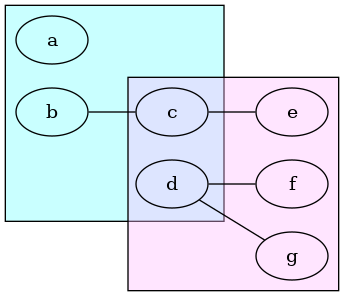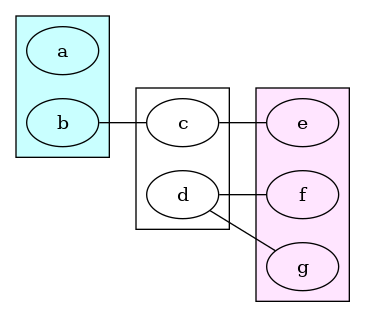# Adding node between two clusters

Is there a way to add node as common between two clusters ? Sample is given in the attached diagram.

If blue is one cluster and brown is another one. I am looking to have node C and D common to both clusters.

Short answer: No, sorry. None of the engines supports overlapping clusters.

With a bit of work answer (if you want some more challenge in your life):

1. Can you create a resulting graph with the nodes and edges placed where you want them? (Using any layout engine, any number of clusters, any fiddling). (Don’t worry about cluster surround/peripheries yet)
2. If you can get the nodes and edges where you want them, then maybe you can modify the graph to build what you want.

The result of roughly an hour of fiddling:First, the starting place - using 3 clusters:

``````graph V{
rankdir=LR
subgraph cluster1{
graph [style=filled fillcolor="#a0ffff90"]
a
b
}
subgraph cluster2{
c d
}
subgraph cluster3{
graph [style=filled fillcolor="#ffbbff60"];
e f g
}
b -- c -- e
d -- f
d --g
}
``````

Giving:Then `dot myfile.gv > myfile.dot` - produces input plus positioning of all nodes, edges & clusters.

Finally, I hand-edited the file (fifteen time or so) to remove the 2nd cluster, move those nodes into the 1st cluster, and resized the bb (bounding boxes) of the two remaining clusters, producing this:

``````graph V {
graph [bb="0,0,266,230",
rankdir=LR
];
node [label="\N"];
subgraph cluster1 {
graph [OLDbb="8,116,78,222"
bb="8,60,172,222" style=filled fillcolor="#a0ffff90"];
a	[height=0.5,
pos="43,196",
width=0.75];
b	[height=0.5,
pos="43,142",
width=0.75];

c	[height=0.5,
pos="133,142",
width=0.75];
d	[height=0.5,
pos="133,88",
width=0.75];
}
subgraph cluster3 {
graph [OLDbb="188,8,258,168"
bb="100,8,258,168" style=filled fillcolor="#ffbbff60"];
e	[height=0.5,
pos="223,142",
width=0.75];
f	[height=0.5,
pos="223,88",
width=0.75];
g	[height=0.5,
pos="223,34",
width=0.75];
}
b -- c	[pos="70.403,142 81.541,142 94.48,142 105.62,142"];
c -- e	[pos="160.4,142 171.54,142 184.48,142 195.62,142"];
d -- f	[pos="160.4,88 171.54,88 184.48,88 195.62,88"];
d -- g	[pos="153.53,76.016 168.11,67.067 187.95,54.892 202.52,45.951"];
}
``````

Finally to produce a finished graph `neato -n2 myfile.dot -Tpng >myfile.png` (see FAQ | Graphviz)